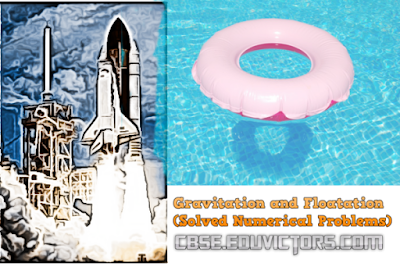## CBSE Class 9 - Physics -Gravitation and Flotation (Solved Numerical Problems)

Questions:

1) Find the total thrust acting on the bottom surface of a tank 4m long, 2m broad and 2m deep when fully filled with water. The density of water= 10³ kg/m³.

2) The gravitational force between two objects is 100N. How should the distance between the objects be changed so that force between them becomes 50N?

3) If the distance between two masses be increased by a factor of 6, by what factor would the mass of one of them have to be altered to maintain the same gravitational force? Would this be an increase or decrease in the mass?

4) The value of ‘g’ on earth’s surface is 9.8 m/s². Suppose the earth suddenly shrinks to one-third of its present size without losing any mass. What is the value of ‘g’ on the surface of shrunk earth?

5) Two bodies of mass 1kg and 100kg respectively are dropped simultaneously from same height 4.9m in a vacuum. Calculate and compare their final velocities just before hitting the ground and the time interval in which they will hit the ground.

6) The ratio of gravitational force on Neptune to the gravitational force on earth is 9:8. Ramesh weighs 792N on earth. Calculate his weight on Neptune.

7) A steel needle sinks in water but a steel ship floats. Explain how?

8) A solid weighs 80g in air and 64g in water. Calculate the relative density of solid. When kept in water, state if the object would float or sink?

9) A body floats in kerosene of density 0.8 × 10³ kg/m³ up to a certain mark. If the same body is placed in water of density 1.0 × 10³ kg/m³, will it sink more or less? Give reasons for your answer.

10) If the radius of the earth becomes half of its present value and its mass becomes four times of its present value, then how would the weight of an object on the surface of the earth be affected?

11) Two bodies of masses 3kg and 12kg are placed at a distance 12m. The third body of mass 0.5kg is to be placed at such a point that the force acting on this body is zero. Find the position of that point.

12) Two stones A and B are dropped from a multi-storeyed building. A is dropped from 100m and after some time B is dropped from a 50m height. Both of them reach the earth at the same time. Will they have equal velocity while reaching the ground? Calculate and find out the answer. (take g=10m/s²)

13) An apple is dropped from the top of a multi-storey building. At the same instant, an arrow is shot vertically upwards from the ground with velocity 11m/s. The arrow hits the apple after 2s. Calculate the height of the building.

14) A cube of side 5cm is immersed in water and then in saturated salt solution. In which case will it experience a greater buoyant force? If each side of the cube is reduced to 4cm and then immersed in water, what will be the effect on the buoyant force experienced by the cube as compared to the first case for water? Give a reason for each case.

15) A ball weighing 4kg of density 4000kg/m³ is completely immersed in water of density 103 kg/m3. Find the force of buoyancy on it. (g=10m/s²)

Solutions: